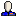chipuni asks how to permute a sequence of natural numbers in order to minimize the sum of pairwise adjacent products. Most of the responses suggest interleaving the sorted sequence of the $$n/2$$ smallest values with the reverse-sorted sequence of the $$n/2$$ largest values, butnicodemusrat's response suggests that it's not quite so trivial...

geomblog:
2005-11-17T08:31:22Z

interesting. nicodemusrat's soln sounds plausible enough (the second one). do you see a proof ?

11011110:
2005-11-17T08:47:13Z

Actually, my reaction to seeing livejournal user nicodemusrat's comment was to start thinking that the problem could be quite difficult. If the most obvious greedy algorithm doesn't work, it seems unlikely that tweaking it a little like that should work any better...

11011110: Proof strategy
2005-11-17T21:03:23Z

I'm now thinking livejournal user nicodemusrat may have an optimal strategy. Proof idea:

Without loss of generality, the largest item must be first. For, if not, you can get a better sequence by reversing the subsequence between the first item and the largest item. By the same sort of flipping argument, the second largest item must be last, the smallest item must be next to the largest, the second smallest must be next to the second largest, etc etc.

Details completely un-worked-out.

geomblog: Re: Proof strategy
2005-11-17T22:12:24Z

In fact, the following is a formal rendition of flipping:

given $$a\lt b\lt c\lt d$$,
$$ad + bc\lt ac + bd$$

in other words, it is always better to "nest" the jumps (ad + bc) rather than interleave them (ac + bd).

I think this is almost sufficient. Going back to the longest path formulation, the expression to be maximized is $$\sum (a_i-a_{i+1})^2 + a_1^2 + a_n^2$$. Now we can pretend that $$0$$ is a special element, and so the last two terms are merely the squared length of arcs from $$a_1$$ and $$a_n$$ to $$0$$.

Now applying the "no interleave rule", we clearly need to start at the largest element (creating a large arc that the rest of the tour nests in). Then we have to jump to the first element (so as to avoid interlocking). We can't then jump to the second largest, because since ultimately we have to return to $$0$$, that would create another interlocking, so we go to the next largest, and then so on.

geomblog: Re: Proof strategy 2005-11-17T22:32:41Z

in fact the above technique is exactly right and can be made precise. the trick is to grow both ends of the "loop" that ends in $$0$$. Or to state another way, grow the tour from $$a_1$$ and $$a_n$$ at the same time. the argument works nicely then.

11011110: Re: Proof strategy 2005-11-17T23:28:28Z

Yes, I think that's another equivalent way of looking at it and somewhat cleaner. I left a comment back at the original post pointing to our discussion here.

geomblog: 2005-11-17T16:05:49Z

an interesting transform is this: if we compute $$\sum (a_i - a_{i+1})^2$$, then the sum is $$C - a_1^2 - a_n^2 -{}$$(the sum of products) so minimizing the pairwise sum (since guessing the start and end points is a poly choice) is equivalent to maximising the pairwise $$l^2_2$$, i.e longest path on this distance function.

geomblog: 2005-11-17T16:07:44Z

hmm. my previous comment got nuked I think. i was saying that this problem is equivalent to longest path on the line with the distance function being $$l^2_2$$: maximising the latter minimizes the former. It explains the heuristics more cleanly

chipuni: 2005-11-18T04:52:58Z

Wow. This is serious.

I had thought that the optimal solution would be found through a dynamic programming strategy. It seems closely related to solving the multiple-matrix multiplication problem.

But I wasn't sure how to go from an optimal series $$a_1, a_2, \dots, a_n$$ to adding another element. I'm not sure that just adding $$a_{n+1}$$ to all possible places in the series, then taking the minimum product-sum, would end up giving an optimal product-sum. (I'm neither denying it, nor accepting it.)

This weekend, I hope that I'll have time to try a few algoritms, and see what works...